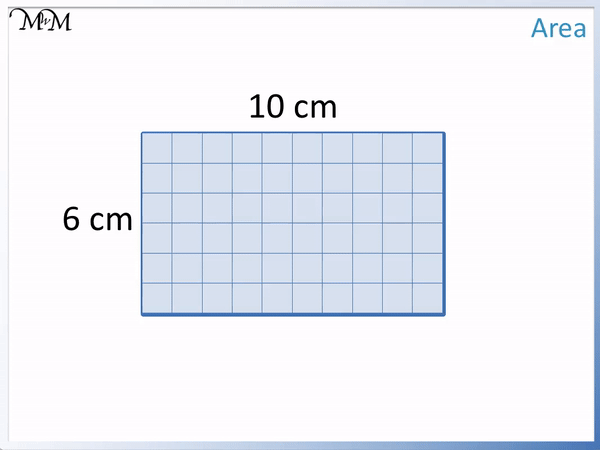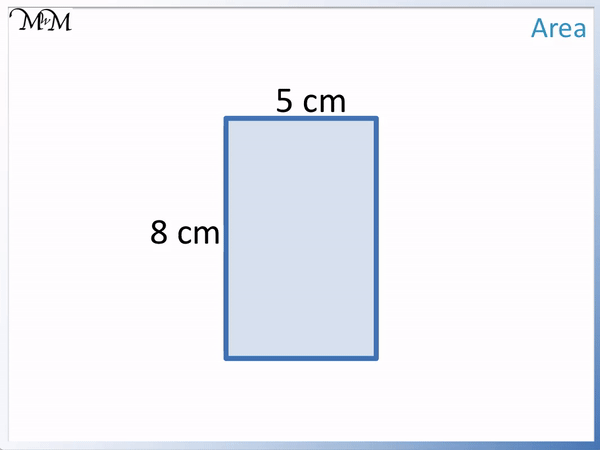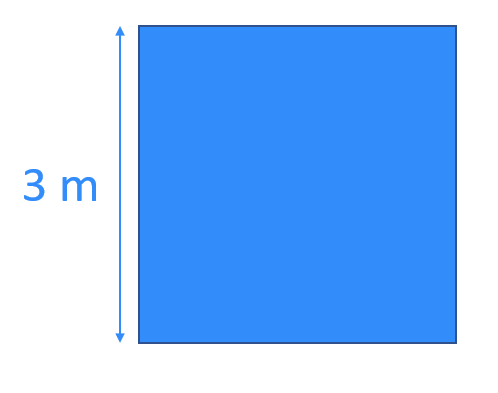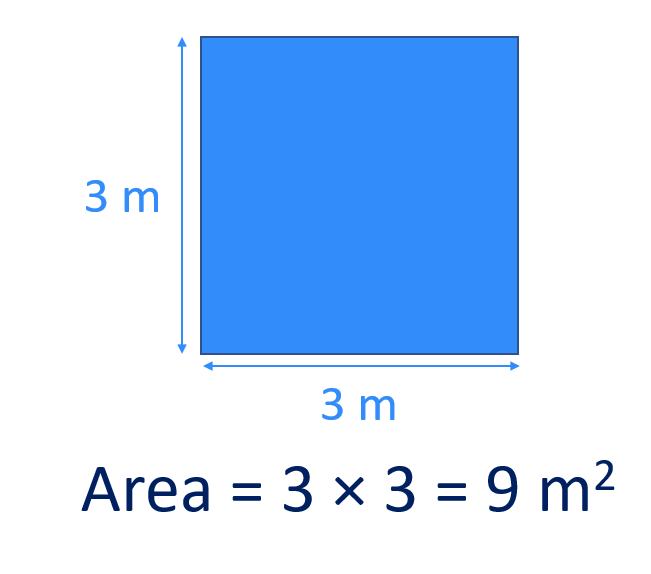# How to Find the Area of a Rectangle

How to Find the Area of a Rectangle• The area of a rectangle is found by multiplying the length by the width.
• The length of a rectangle is the longest side length.
• The width of a rectangle is the shortest side length.
• The area of this rectangle is 6 cm × 10 cm = 60 cm2.
• The area of a rectangle tells us how many unit squares can fit inside it.
• We have 6 rows of 10 squares, which is 60 squares in total.
• Area is measured in units squared.
• Because we have measured the sides in centimetres, the area is measured in centimetres squared.
The area of a rectangle is length × width.

Area is measured in units squared.• The area of a rectangle is found by multiplying the length and width.
• The length is 7 m and the width is 3 m.
• The area of the rectangle is 3 mm × 7 mm = 21 mm2.
• This means that 21 individual 1 mm2 squares can fit inside the rectangle.Supporting Lessons# Finding the Area of Rectangles and Squares

## How to Find the Area of a Rectangle

To find the area of a rectangle, multiply its length by its width. The area is measured in units squared. For example, for a rectangle of length 8 cm and width 5 cm, the area is 8 cm × 5 cm = 40 cm2.The length of a rectangle is the longest side and the width is the shortest side.

In this example, the length is 8 cm and the width is 5 cm.

8 × 5 = 40 and the units for the area of this rectangle are cm2. The area of this rectangle is 40 cm2.

We multiply 8 cm by 5 cm. Multiplying cm × cm gives us cm2.

The formula for the area of a rectangle is Area = Length × Width. This formula can be written as A = L × W or as A = LW.

Here is an example of using the formula to calculate the area of a rectangle.Area = Length × Width.

Length = 9 cm and Width = 4.

A = 9 × 4 = 36. The units for the area are cm2 since we measured the sides in centimetres.

The area of this rectangle is 36 cm2.

Here is a word problem for finding the area of a rectangle.The area is found by multiplying the length and the width. In this worded problem, we just need to find the two numbers that show the length and width.

L = 7 and W = 5.

Area = 7 × 5 = 35. The units are cm2. The area of the rectangle is 35 cm2.

## How to Find the Area of a Square

To find the area of a square, multiply the length of one side by itself. The units for area are units squared. For example, if a square has a side length of 3 m, then its area is 3 m × 3m = 9 m2.

Here is a square with a side length of 3 m.A square is a special type of rectangle that has all of its sides the same length.

Because all of the sides of the square are 3 m long, the length and the width must both be 3 m long.Multiplying the length by the width, the area is 3 m × 3m = 9 m2.

Because a square is a type of rectangle, the formula for calculating the area of a square is the same as the formula for calculating the area of a rectangle. We can use length × width. However, the length and the width are both the same on a square, which makes it even easier to find the area.

The formula for the area of a square is Area = Side Length × Side Length. This formula can be written more simply as Area = L2, where L is the length of one side of the square.

Here is an example of using the formula for the area of a square with a worded problem.The area of a square is found by Area = Side Length × Side Length. The side length = 6 cm. We simply multiply 6 by 6.

6 cm × 6 cm = 36 cm2 and so, the area of this square is 36 cm2.

Using the alternative form of the formula, we have Area = L2.

L2 means to multiply the value of L by itself.

L = 6 cm and so, L2 = 6 × 6 = 36.

The area is 36 cm2.

## Why is the Area of a Rectangle Length Times Width?

The area of a rectangle is the number of unit squares that fit inside it. The length is the number of unit squares that fit in each row and the width is the number of rows of squares. Multiplying the length by the width gives the total number of unit squares inside a rectangle and hence, the area.

Measuring the length or width in a given unit of measurement tells us how many of these units can fit in this direction.

In this example we will use centimetre squares.Inside the rectangle we can look at individual squares. These squares measure 1 cm by 1 cm. Their area is 1 cm2.

To find the total area of the rectangle, we simply need to count the number of 1 cm2 squares that fit inside it.

Here is a rectangle that is 10 cm long and 6 cm wide.

We can see that there are 10 of the unit squares that can fit in each row because the side length is 10 cm long.We can see that there are 6 rows because the width is 6 cm long.We have 6 rows of 10 or 6 lots of 10.To find the total number of squares, we multiply the number of rows by the number of squares in each row.

6 lots of 10 is written as 6 × 10, which equals 60 squares.

Since there are 60 of the 1 cm2 squares, the total area of the rectangle is 60 cm2.Now try our lesson on How to Find the Area of a Triangle where we learn how to find the area of a triangle.